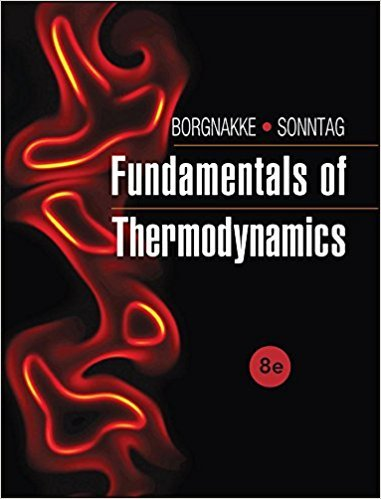×
×

# Nozzles, DiffusersA nozzle receives an ideal gas flow withISBN: 9781118131992 58

## Solution for problem 21HP Chapter 4

Fundamentals of Thermodynamcs | 8th Edition

• Textbook Solutions
• 2901 Step-by-step solutions solved by professors and subject experts
• Get 24/7 help from StudySoup virtual teaching assistantsFundamentals of Thermodynamcs | 8th Edition

4 5 1 298 Reviews
30
3
Problem 21HP

Nozzles, Diffusers

A nozzle receives an ideal gas flow with a velocity of 25 m/s, and the exit at 100 kPa, 300 K velocity is 250 m/s. Determine the inlet temperature if the gas is argon, helium, or nitrogen.

Step-by-Step Solution:

Solution 21 HP

Step 1 of 5</p>

We need to find the inlet temperatures of Argon, Helium and Nitrogen

Step 2 of 5</p>

Energy equation is given byJ/kgkJ/kg

Step 3 of 5</p>

We get specific heats of ideal gases from table A.5kJ/kg.K for argonUse the formula to find inlet temperatureK

Inlet temperature of Argon gas isK

Step 4 of 5

Step 5 of 5

##### ISBN: 9781118131992

This textbook survival guide was created for the textbook: Fundamentals of Thermodynamcs , edition: 8. Since the solution to 21HP from 4 chapter was answered, more than 513 students have viewed the full step-by-step answer. The full step-by-step solution to problem: 21HP from chapter: 4 was answered by , our top Engineering and Tech solution expert on 08/03/17, 05:05AM. The answer to “Nozzles, DiffusersA nozzle receives an ideal gas flow with a velocity of 25 m/s, and the exit at 100 kPa, 300 K velocity is 250 m/s. Determine the inlet temperature if the gas is argon, helium, or nitrogen.” is broken down into a number of easy to follow steps, and 38 words. This full solution covers the following key subjects: velocity, gas, inlet, exit, flow. This expansive textbook survival guide covers 7 chapters, and 1462 solutions. Fundamentals of Thermodynamcs was written by and is associated to the ISBN: 9781118131992.

Unlock Textbook Solution